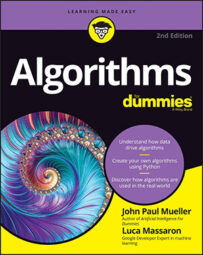##### Algorithms For DummiesThe more operations an algorithm requires, the more complex it is. Complexity is a measure of algorithm efficiency in terms of time usage because each operation takes some time. Given the same problem, complex algorithms are generally less favorable than simple algorithms because complex algorithms require more time.

Think about those times when speed of execution makes the difference, such as in the medical or financial sector, or when flying on automatic pilot on an airplane or space rocket. Measuring algorithm complexity is a challenging task, though a necessary one if you want to employ the right solution. The first measurement technique uses abstract machines like the Random Access Machine (RAM).

RAM also stands for Random-Access Memory, which is the internal memory that your computer uses when running programs. Even though it uses the same acronym, a Random-Access Machine is something completely different.

Abstract machines aren't real computers, but theoretical ones, computers that are imagined in their functioning. You use abstract machines to consider how well an algorithm would work on a computer without testing it on the real thing, yet bound by the type of hardware you'd use. A RAM computer performs basic arithmetic operations and interacts with information in memory, that's all. Every time a RAM computer does anything, it takes a time step (a time unit). When you evaluate an algorithm in a RAM simulation, you count time steps using the following procedure:
1. Count each simple operation (arithmetic ones) as a time step.
2. Break complex operations into simple arithmetic operations and count time steps as defined in Step 1.
3. Count every data access from memory as one time step.
To perform this accounting, you write a pseudocode version of your algorithm and perform these steps using paper and pencil. In the end, it's a simple approach based on a basic idea of how computers work, a useful approximation that you can use to compare solutions regardless of the power and speed of your hardware or the programming language you use.

Using a simulation is different from running the algorithm on a computer because you use a standard and predefined input. Real computer measurements require that you run the code and verify the time required to run it. Running code on a computer is actually a benchmark, another form of efficiency measurement, in which you also account for the application environment (such as the type of hardware used and the software implementation). A benchmark is useful but lacks generalization. Consider, for instance, how newer hardware can quickly execute an algorithm that took ages on your previous computer.